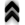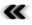### 5.1 Newton’s Mechanics and World

Drawing on considerations and abstracts of thinkers such as Thomas Aquinas and Descartes Newton was essential in formulating the stage from which to arrive at the abstraction of phase space. To this end it is important to recall that Newton formulates his famous three laws or axioms of motion:

• “Corpus omne perseverare in statu suo quiescendi vel movendi uniformiter in directum, nisi quatenus a viribus impressis cogitur statum illum mutare” – a body remains in a state of rest or uniform motion unless a force acts upon it.
• “Mutationem motus proportionalem esse vi motrici impressæ, & ﬁeri secundum lineam rectam qua vis illa imprimitur” – the change of a motion is proportional to and at a right angle to the impressed force.
• “Actioni contrariam semper & æqualem esse reactionem: sive corporum duorum actiones in se mutuo semper esse æquales & in partes contrarias dirigi” – to every action there is always an equal action opposed to it: when two bodies act on each other, their actions are always equal and in opposition to each other.

He thus formulated the motion of bodies (or particles) focusing on the concept of forces which are set in a space using an absolute time which is mathematically expressed as an aﬃne space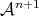(Deﬁnition 79)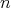stands for the degrees of freedom and the additional dimension is added to accommodate time. The absolute nature of time is modelled as a scalar ﬁeld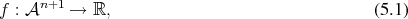which assigns the time to each point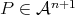(symbolizing an event).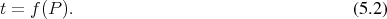The diﬀerential (Deﬁnition 70) of this scalar ﬁeld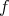(Deﬁnition 61),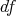is constant in correspondence to the absolute nature of time in Newton’s setting and deﬁnes a mapping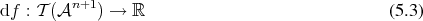of the tangent space(Deﬁnition 48), which determines time spans between two events. Furthermore, the kernel (Deﬁnition 22) of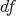is an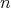-dimensional Euclidean (Deﬁnition 80) subspace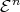of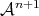corresponding to all simultaneously occurring events. An aﬃne space with this additional structure is called a Galilean space . A Galilean space has a natural ﬁber bundle (Deﬁnition 40) structure of a one-dimensional base space (time), with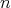-dimensional ﬁbers (space) as is illustrated in Figure 5.1.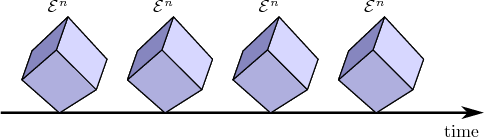Figure 5.1: Fiber structure of a Galilean space.

Each of the ﬁbers contains events which are said to occur at the same time. The evolution of an entity in the aﬃne space with the passing of time is represented as a section of this ﬁber bundle. While it is simple to naturally denote a one-dimensional base space, with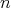-dimensional ﬁbers, the reverse is not the case, as while time is deﬁned as absolute, thusly globally deﬁning simultaneous events and thus ﬁxed points in time, it is not clear what ﬁxed points in space should be.

A transformation, which leaves the structure of a Galilean space invariant, is called a Galilean transformation. Coordinate systems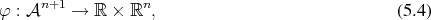in which Newton’s axioms hold parametrize the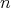-dimensional subspaces and are called inertial. Galilean transforms provide mappings between diﬀerent inertial systems.

In this setting forces and the connected accelerations may be expressed as functions of the form: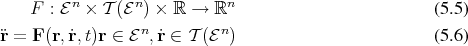A system is determined uniquely by specifying the initial conditions at time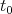for the position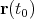and the velocity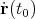. Together with Newton’s three laws of motion a multitude of problems can already be considered.

The relevance of Galilean transfoms is connected to the evolution of physical quantities in the system. The laws how to determine (measure) a quantity do not depended on time, thus the evolution of quantities is described by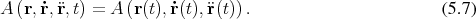As Galilean transforms do not interfere with this property, they are of special interest.

However, the formalism has been developed within the Cartesian setting of aﬃne spaces. This leads to complications as the problems to which the formalism is applied increases in sophistication and adds complexity by being formulated directly in coordinate expressions. This leads to a strong mix of the representation in coordinates and the physical content, which is independent from the choice of coordinates. The search to overcome these limitations leads to a generalized reformulation of classical mechanics in the form of Lagrangian mechanics which recasts the single second order equation as two ﬁrst order equations.